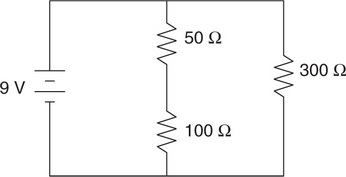# AP Physics 1 Question 154: Answer and Explanation

### Test Information

Question: 154

4.Question below refers to the circuit shown in the figure, which includes a 9 V battery and three resistors.

Which of the following ranks the resistors by the charge that flows through each in a given time interval?

• A. 300 Ω > 100 Ω = 50 Ω
• B. 50 Ω > 100 Ω > 300 Ω
• C. 300 Ω > 100 Ω > 50 Ω
• D. 50 Ω = 100 Ω > 300 Ω